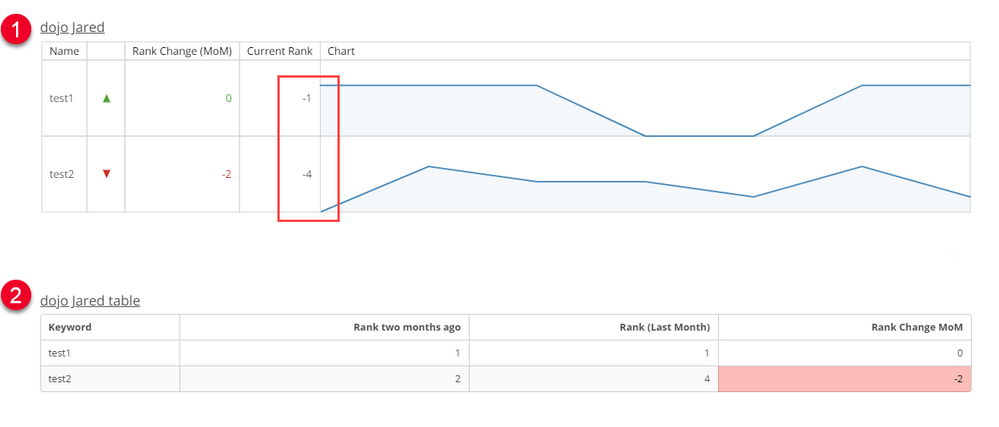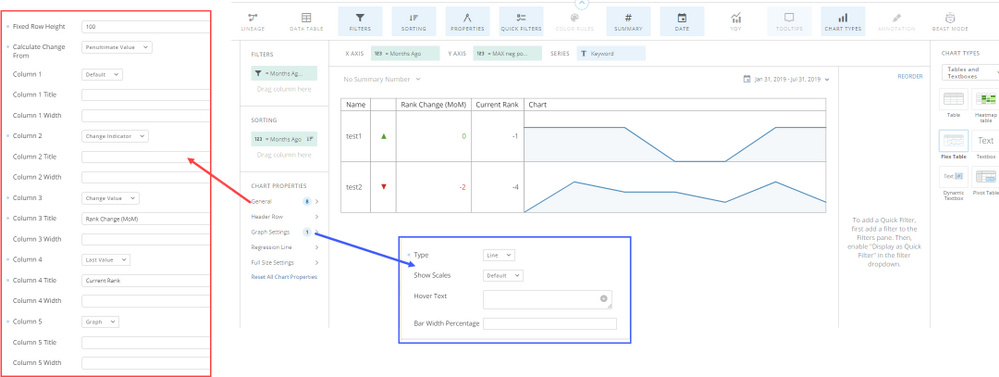# MoM formula in table card

Hi,

I trying to create a table chart for keyword rankings.

The chart will have 3 columns: Keyword, Rank(Last Month), Rank Change (MoM).

I am having trouble showing this in a table chart format. If the table is only supposed to show last month's Position, it filters out other dates - so how would the Rank Change MoM be calculated? The only way I managed to do this is by Uncollapsing Columns in ETL and moving dates in Columns, but this requires manual adjustment every month so it is not automated.

Some formulas I am using:

Rank (Last Month):

case when MONTH(`Date`)=MONTH(CURDATE())-1 then `Position` end

Rank Change MoM:

case when YEAR(CURDATE())=year(`Date`) AND MONTH(`Date`)=(MONTH(CURDATE())-1) then `Position` end
-
case when YEAR(CURDATE())=year(`Date`) AND MONTH(`Date`)=(MONTH(CURDATE())-2) then `Position` end

Below is an example of raw data:

 Keyword Position Date test1 1 6/1/19 test1 3 5/31/19 test1 3 4/1/19 test1 1 3/31/19 test1 1 2/1/19 test1 1 1/31/19 test1 1 7/31/19 test2 2 6/1/19 test2 4 5/31/19 test2 3 4/1/19 test2 3 3/31/19 test2 2 2/1/19 test2 5 1/31/19 test2 4 7/31/19

Does anyone have a suggestion on how to make this possible, or if I am doing somehting wrong? thank you!

• I see two ways to display this data.  I'll try to explain both so you can pick which you prefer.

The first option is to create a flex table.  The second option is to leave it in a regular table.The main issue with the flex table is that you can't tell the table the low values are good.  This means that in order to have the change indicator work correctly, you need to multiply your `Position` field by neg 1.  This, in turn, makes the current rank have a negative value.  However, I like the visibility that the flex table offers to view performance over time.  Another issue with this card is that the two scales do not sync.  Meaning that the lowest value for "test1" is rank 3 but it appears at the bottom of the graph so it appears to have the same value as "test2"s lowest value of rank 5.  The same effect is true for the highest values.  (while test1 has been rank 1, the graph represents that value to appear the same as rank 2 for test2).

To make the flex table I had to create two beastmodes.

`-- Months Ago:case 	when year(DATE_SUB(CURDATE(), interval 1 MONTH)) = YEAR(`Date`) 	and MONTH(DATE_SUB(CURDATE(), interval 1 MONTH)) = MONTH(`Date`)    	THEN 1    when year(DATE_SUB(CURDATE(), interval 2 MONTH)) = YEAR(`Date`) 	and MONTH(DATE_SUB(CURDATE(), interval 2 MONTH)) = MONTH(`Date`)    	THEN 2    when year(DATE_SUB(CURDATE(), interval 3 MONTH)) = YEAR(`Date`) 	and MONTH(DATE_SUB(CURDATE(), interval 3 MONTH)) = MONTH(`Date`)    	THEN 3    when year(DATE_SUB(CURDATE(), interval 4 MONTH)) = YEAR(`Date`) 	and MONTH(DATE_SUB(CURDATE(), interval 4 MONTH)) = MONTH(`Date`)    	THEN 4    when year(DATE_SUB(CURDATE(), interval 5 MONTH)) = YEAR(`Date`) 	and MONTH(DATE_SUB(CURDATE(), interval 5 MONTH)) = MONTH(`Date`)    	THEN 5    when year(DATE_SUB(CURDATE(), interval 6 MONTH)) = YEAR(`Date`) 	and MONTH(DATE_SUB(CURDATE(), interval 6 MONTH)) = MONTH(`Date`)    	THEN 6    when year(DATE_SUB(CURDATE(), interval 7 MONTH)) = YEAR(`Date`) 	and MONTH(DATE_SUB(CURDATE(), interval 7 MONTH)) = MONTH(`Date`)    	THEN 7    when year(DATE_SUB(CURDATE(), interval 8 MONTH)) = YEAR(`Date`) 	and MONTH(DATE_SUB(CURDATE(), interval 8 MONTH)) = MONTH(`Date`)    	THEN 8    when year(DATE_SUB(CURDATE(), interval 9 MONTH)) = YEAR(`Date`) 	and MONTH(DATE_SUB(CURDATE(), interval 9 MONTH)) = MONTH(`Date`)    	THEN 9    when year(DATE_SUB(CURDATE(), interval 10 MONTH)) = YEAR(`Date`) 	and MONTH(DATE_SUB(CURDATE(), interval 10 MONTH)) = MONTH(`Date`)    	THEN 10    when year(DATE_SUB(CURDATE(), interval 11 MONTH)) = YEAR(`Date`) 	and MONTH(DATE_SUB(CURDATE(), interval 11 MONTH)) = MONTH(`Date`)    	THEN 11    when year(DATE_SUB(CURDATE(), interval 12 MONTH)) = YEAR(`Date`) 	and MONTH(DATE_SUB(CURDATE(), interval 12 MONTH)) = MONTH(`Date`)    	THEN 12end`

and

`-- Neg Position:-1*`Position``For the table, I created three beastmodes:

1

`-- Rank (Last Month)max(case     when year(DATE_SUB(CURDATE(), interval 1 MONTH)) = YEAR(`Date`) 	and MONTH(DATE_SUB(CURDATE(), interval 1 MONTH)) = MONTH(`Date`)     then `Position`     end)`

2

`-- Rank (two months ago)max(case     when year(DATE_SUB(CURDATE(), interval 2 MONTH)) = YEAR(`Date`) 	and MONTH(DATE_SUB(CURDATE(), interval 2 MONTH)) = MONTH(`Date`)     then `Position`     end)`

3

`-- Rank (two months ago)max(case     when year(DATE_SUB(CURDATE(), interval 2 MONTH)) = YEAR(`Date`) 	and MONTH(DATE_SUB(CURDATE(), interval 2 MONTH)) = MONTH(`Date`)     then `Position`     end)--- Rank (Last Month)max(case     when year(DATE_SUB(CURDATE(), interval 1 MONTH)) = YEAR(`Date`) 	and MONTH(DATE_SUB(CURDATE(), interval 1 MONTH)) = MONTH(`Date`)     then `Position`     end)`

The advantage here is that you can easily control which direction is negative.  However, without adding a seperate beastmode for every month, you won't see performance over time beyond the last two months.

A few notes on the changes I made to the beastmode calculations:

When you calculate last month, you were simply using when MONTH(`Date`) = MONTH(CURDATE())-1

You should consider how that will calculate in January.  By changing it to compare the year and month of `Date` to the actual date one month ago (DATE_SUB(CURDATE(), interval 1 MONTH) you will not have to worry about this calculation at the beginning of the year.

I also chose to aggregate the rank beastmodes.  This is because you have a row of data for each month, for each user.  By adding MAX() to the case statement, it will look through all rows for that "keyword" and give you the max value.  However, since all of the rows will be null except for the the one row for the month we are concerned with, you could really use any aggregate function ( MIN, SUM, etc.) ... just not COUNT.

Hope that helps.  Let me know if you have any questions.

______________________________________________________________________________________________
“There is a superhero in all of us, we just need the courage to put on the cape.” -Superman
______________________________________________________________________________________________